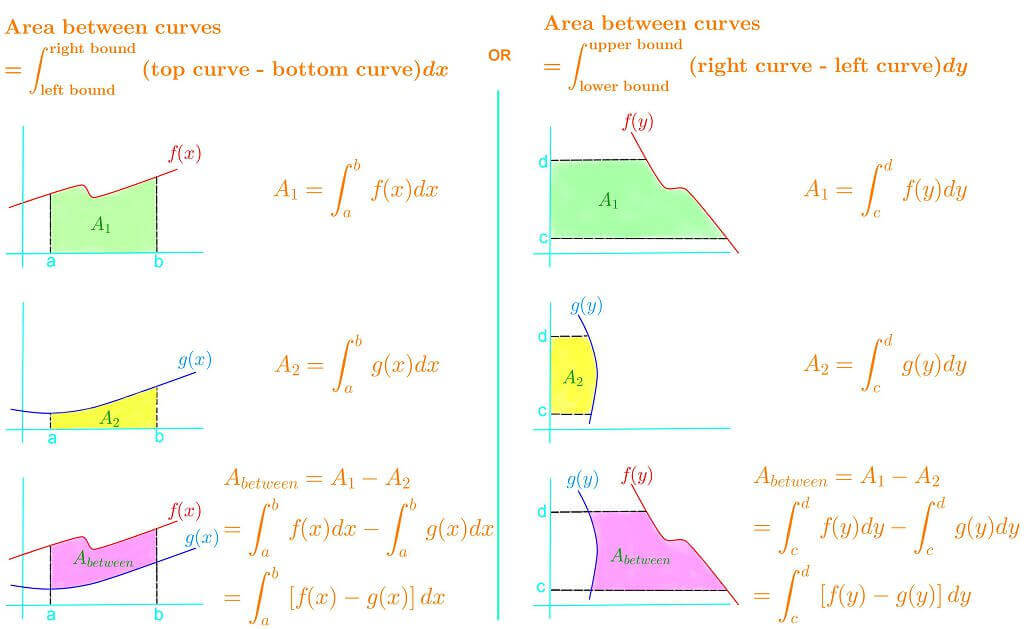# Areas between curves

### Areas between curves

So we learn that we can find the area under the curve, but we can actually find the area between two curves by taking the difference between the top curve and bottom curve, and integrating it in terms of x! Just make sure to pick your lower and upper bound correctly so that you are actually finding the area you are looking for. However, there may be cases where you don't really know which is the top curve and which is the bottom curve. In this case, you would instead have a left curve and right curve. To find the area between, you would take the difference between the right curve and the left curve and integrate in terms of y. In this section, we will take a look at all of these cases and write the integral correctly.

#### Lessons

Note:• Introduction

• 1.
Find the area of the region bounded between $y=2x-1$ and $y=1+e^x$ and bounded on the sides by $x=0$, and $x=2$.

• 2.
a)
Find the area of the region enclosed by the parabolas $y=(x-3)^2$ and $y=-x^2+8x-15$

b)
Find the area of the region bounded by $y=(x-3)^2$, $y=-x^2+8x-15$, $x=2$ and $x=5$

• 3.
Find the area enclosed by $y=2x+4$ and $y^2=11+4x$